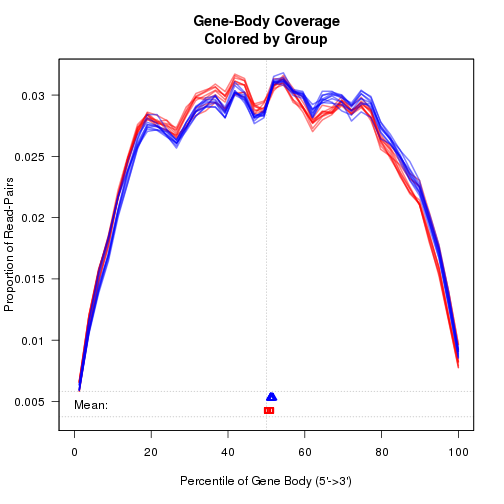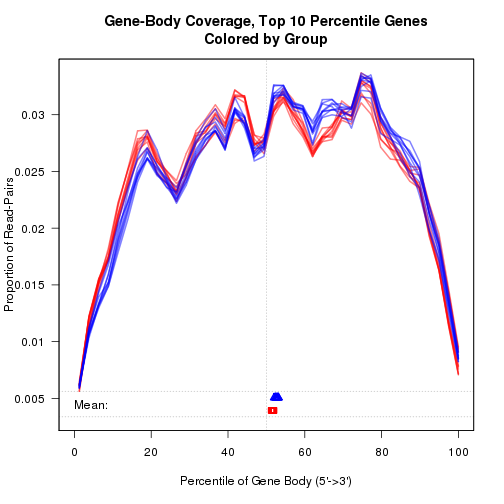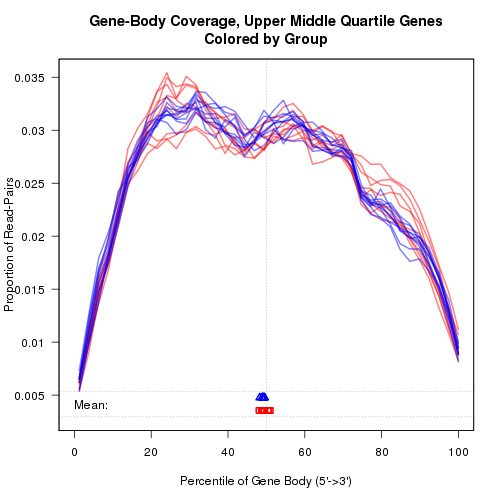makePlot.genebody.coverage {QoRTs} R Documentation

## Plot Gene-Body coverage distribution

### Description

Plots Gene-Body coverage distribution

### Usage

```  makePlot.genebody(plotter,
geneset = c("Overall","90-100","75-90","50-75","0-50"),
avgMethod = c("TotalCounts", "AvgPercentile"),
plot.medians,
plot.means = TRUE,
debugMode,
singleEndMode,
rasterize.plotting.area = FALSE, raster.height = 1000, raster.width = 1000,
plot = TRUE,
... )

#NOTE: The preferred method to access the below functions
#  is to use makeplot.genebody and set:
#  avgMethod = "TotalCounts"
#DEPRECIATED:
makePlot.genebody.coverage(plotter, plot.medians,
plot.means = TRUE,
debugMode, singleEndMode,
rasterize.plotting.area = FALSE, raster.height = 1000, raster.width = 1000,
plot = TRUE,
...)
#DEPRECIATED:
makePlot.genebody.coverage.UMQuartile(plotter, plot.medians,
plot.means = TRUE,
debugMode, singleEndMode,
rasterize.plotting.area = FALSE, raster.height = 1000, raster.width = 1000,
plot = TRUE,
...)
#DEPRECIATED:
makePlot.genebody.coverage.lowExpress(plotter, plot.medians,
plot.means = TRUE,
debugMode, singleEndMode,
rasterize.plotting.area = FALSE, raster.height = 1000, raster.width = 1000,
plot = TRUE,
...)
```

### Arguments

 `plotter` A `QoRT_Plotter` reference object. See `build.plotter`. `geneset` The set of genes to plot the gene-body coverage over. Genes are grouped into four quantiles by their by their total read counts: the 90-100 quantile, the 75-90 quantile, the 50-75 quantile (or "upper-middle quartile"), and the 0-50 quantile. By default it will plot the overall gene-body coverage across all genes. `avgMethod` The method used to generate average gene body coverage. The "TotalCounts" (default) method simply takes the sum of each bin across all genes in the geneset, then for each replicate normalizes the coverage into frequencies. The "AvgPercentile" calculates the coverage distribution across each percentile bin and normalizes the counts so they add up to 1, FOR EACH GENE, and THEN averages those values across all genes in the geneset. The latter variant is experimental. the idea was for it to reduce the impact of highly-expressed genes and gain a better estimate of the degree of 5'/3' bias. `plot.medians` A logical value indicating whether or not to plot the median average for each bamfile at the bottom of the plot. Overrides `plot.means`. `plot.means` A logical value indicating whether or not to plot the mean average for each bamfile at the bottom of the plot. `debugMode` Logical. If TRUE, debugging data will be printed to the console. `singleEndMode` Logical. Determines whether the dataset consists of single-ended reads. By default this is determined from the data. Thus, this parameter should never need to be set by the user. `rasterize.plotting.area` Logical. If `TRUE`, then "flatten" the plotting lines into a raster format. This requires support for png file creation and the installation of the "png" package. Only the plotting lines will be rasterized, the axes and annotations will be vector format. Default is `FALSE`. `raster.height` Numeric integer. If `rasterize.plotting.area` is `TRUE`, then this will set the height of the rasterized plot, in pixels. `raster.width` Numeric integer. If `rasterize.plotting.area` is `TRUE`, then this will set the width of the rasterized plot, in pixels. `plot` Logical. If FALSE, suppress plotting and return `TRUE` if and only if the data is present in the dataset to allow plotting. Useful to automatically filter out missing data plots. `...` Arguments to be passed to methods, such as graphical parameters (see `par`).

### Details

x-axis: Gene-body quantile. By default this is broken up into 40 quantiles containing 2.5

y-axis: Rate at which reads falls into the given quantile of the genes' bodies.

`makePlot.genebody.coverage` plots the gene body coverage across all genes. `makePlot.genebody.coverage.UMQuartile` plots the gene body coverage across the genes that fall in the upper-middle quartile of total expression for each sample-run (excluding genes with zero reads). `makePlot.genebody.coverage.lowExpress` plots the gene body coverage across the genes that fall in the lower two quartiles of total expression for each sample-run (excluding genes with zero reads).

`build.plotter`

### Examples

``````  data(res,package="QoRTsExampleData");
plotter <- build.plotter.colorByGroup(res);
makePlot.genebody(plotter, geneset = "Overall");
``````
``````## Starting: Gene-Body Coverage plot.
``````
``````## Finished: Gene-Body Coverage plot.[time: 2018-09-25 13:12:34],[elapsed: 0.13 secs]
````````````  makePlot.genebody(plotter, geneset = "90-100");
``````
``````## Starting: Gene-Body Coverage, Top 10 Percentile Genes plot.
``````
``````## Finished: Gene-Body Coverage, Top 10 Percentile Genes plot.[time: 2018-09-25 13:12:34],[elapsed: 0.18 secs]
````````````  makePlot.genebody(plotter, geneset = "50-75");
``````
``````## Starting: Gene-Body Coverage, Upper Middle Quartile Genes plot.
``````
``````## Finished: Gene-Body Coverage, Upper Middle Quartile Genes plot.[time: 2018-09-25 13:12:35],[elapsed: 0.18 secs]
``````[Package QoRTs version 1.3.6 Index]